## Example Questions

### Example Question #5 : Hexagons

The perimeter of a regular hexagon is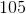. What is the length of one of its diagonals?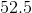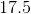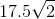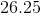Explanation:

To begin, calculate the side length of the hexagon. Since it is regular, its sides are of equal length. This means that a given side is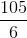orin length. Now, consider your figure like this: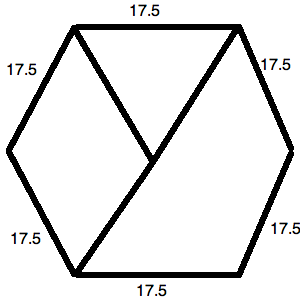The little triangle at the top forms an equilateral triangle. This means that all of its sides are. You could form six of these triangles in your figure if you desired. This means that the long diagonal is really just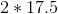or.

### Example Question #6 : Hexagons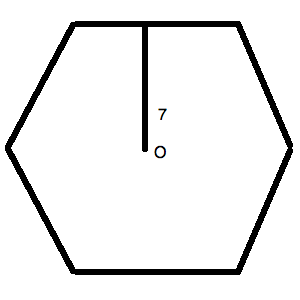The figure above is a regular hexagon.  O is the center of the figure.  The line segment makes a perpendicular angle with the external side.

What is the length of the diagonal of the regular hexagon pictured above?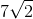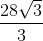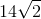Explanation:

You could redraw your figure as follows.  Notice that this kind of figure makes an equilateral triangle within the hexagon.  This allows you to create a useful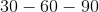triangle.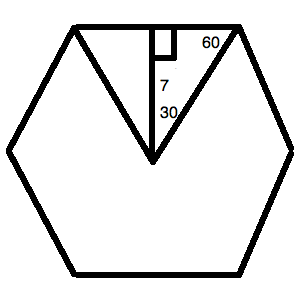Thein the figure corresponds to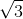in a referencetriangle. The hypotenuse isin the reference triangle.

Therefore, we can say: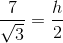Solve for: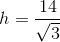Rationalize the denominator: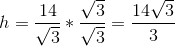Now, the diagonal of a regular hexagon is actually just double the length of this hypotenuse. (You could draw another equilateral triangle on the bottom and duplicate this same calculation set—if you wanted to spend extra time without need!) Thus, the length of the diagonal is: